﻿ Python: Get the smallest number from a list - w3resourcePython: Get the smallest number from a list

Python List: Exercise-4 with Solution

Write a Python program to get the smallest number from a list.

Example - 1 :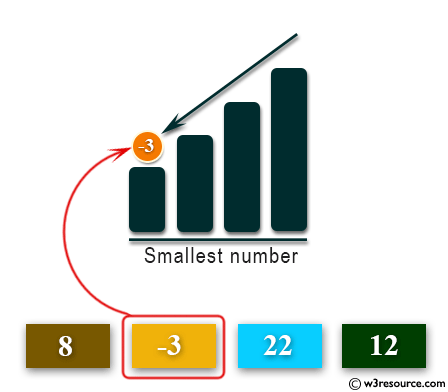Example - 2 :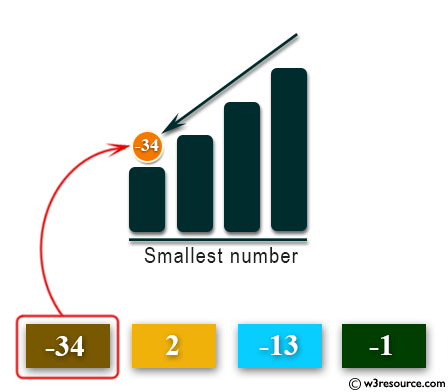Example - 3 :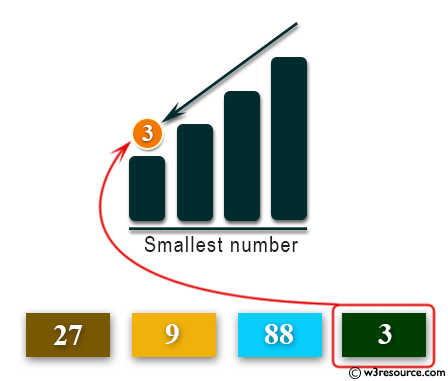Example - 4 :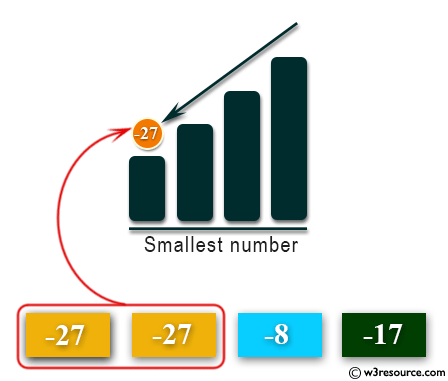Sample Solution:

Python Code:

def smallest_num_in_list( list ):
min = list[ 0 ]
for a in list:
if a < min:
min = a
return min
print(smallest_num_in_list([1, 2, -8, 0]))

Sample Output:

-8

Flowchart: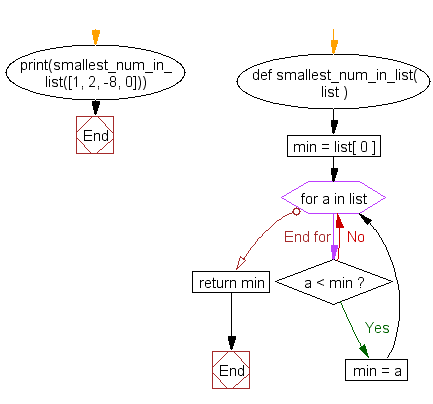Visualize Python code execution:

The following tool visualize what the computer is doing step-by-step as it executes the said program:

Python Code Editor:

Have another way to solve this solution? Contribute your code (and comments) through Disqus.

What is the difficulty level of this exercise?

Test your Programming skills with w3resource's quiz.

﻿

Python: Tips of the Day

Time it:

timeit library is great for timing Python execution times. Just pass a function in string format to timeit.timeit() method and it will carry out 1 million executions to report the minimum time the code takes.

Its very useful to compare small code pieces and different functions but can be sluggish with big code.

Check out the example below demonstrating the execution time difference between 2 very similar list comprehension methods in Python:

import timeit
lst1='''list(range(100))'''
lst2='''[i for i in range(100)]'''
a=timeit.timeit(lst1)
b=timeit.timeit(lst2)
print(a, b, sep="------")

Output:

1.9301698543131351------5.389458132907748